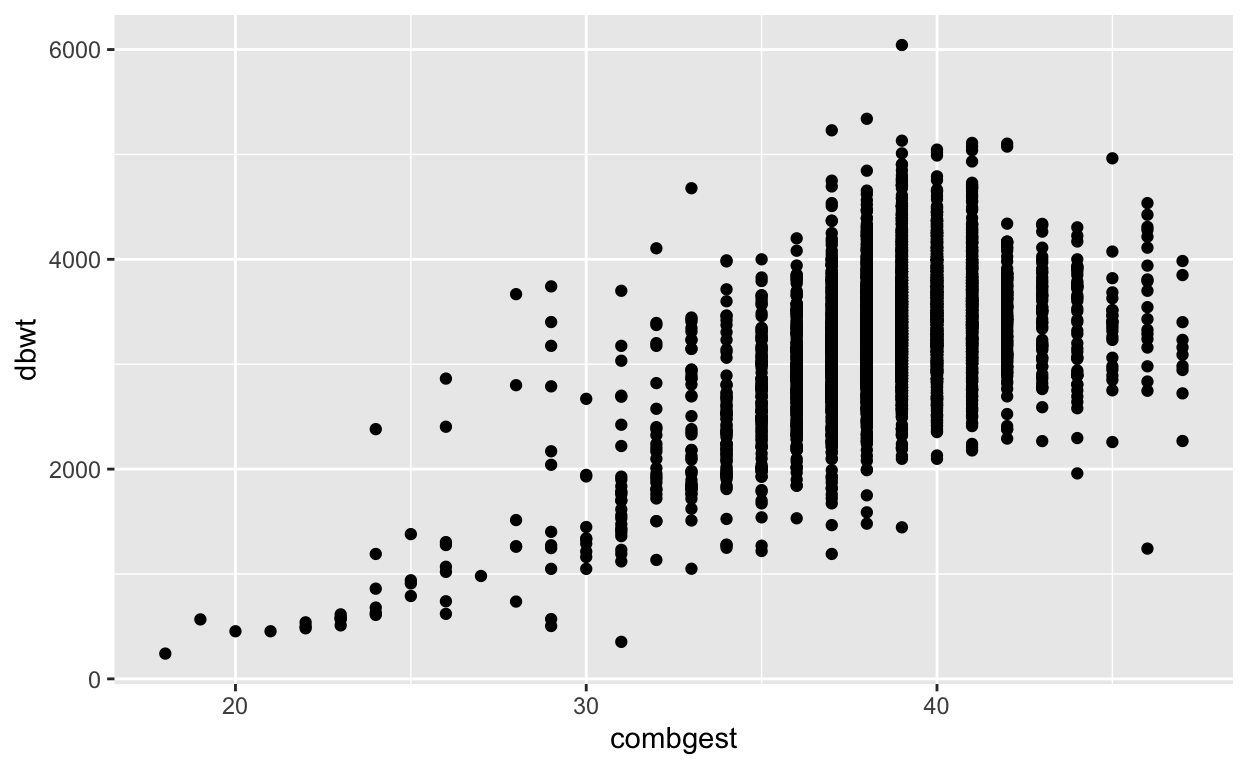# Data and point plots

Introduces the distinction between quantitative and categorical variables through their very different appearances in a point plot.

Daniel Kaplan https://dtkaplan.github.io
2020-06-16

Alternative document formats: Word & PDF

# Orientation

Data can be many things, but one of the most common formats is a data frame, a kind of spreadsheet of rows and columns. We’ll work with the data frame (or data set) Natality_2014 in the Source pakage Little Apps, which is based on data published by the US Centers for Disease Control. Natality_2014 has 100,000 rows. Each row reports a live birth in the US in 2014. There are dozens of variables, a few of which are shown below.

It’s hard to draw much of a conclusion by looking directly at a large data frame. But a graphical display of data can help.

A point plot1 is a basic statistical graphic that displays two variables from a data frame. One variable is represented on the vertical axis, another variable on the horizontal axis. Like the following point plot of the baby’s weight (in grams) (dbwt) and the length (in weeks) of the pregnancy (combgest).# Exercise

Referring to the graph in the previous section …

1. Find in the graph the dot corresponding to the first row in the data table above, the one for a male baby delivered spontaneously to a 28 year-old mother.
2. Describe the overall pattern shown in the graph as a whole. Use whatever form of description you think is appropriate.
3. Of course, weight differs from one baby to another. In other words, weight varies. Describe how much variation there is in babies’ weight, according to the graph.
4. Describe how much variation there is in gestation length.
5. At which length of gestation are the heaviest babies born?

# Activity

Open the Regression Little App. (See footnote2).

1. Set the Source package to Little Apps, data set to Natality_2014. Choose dbwt as the response variable and combgest as the explanatory variable. The resulting plot should look much like the graph seen in the introduction to this lesson. Change the sample size to $$n = 5$$ by cliciking on the n=50 icon and choosing n=5. Click on the Graph tab in the top tool bar to see a larger graph.

2. In the “Data” tab in the top tool bar you will see the graph and the data that is in the plot, in data-frame format.

• For each of the $$n=5$$ rows of the data frame, find the corresponding point in the graphic.*
3. Change the explanatory variable to sex.

• For each of the $$n=5$$ rows of the data frame displayed in the Data tab, find the corresponding point in the graphic.

• Change $$n$$ to 500. In the baby_wt versus sex graph, all the points are lined up in two columns.

Explain why. . . .

Version 0.3, 2020-08-13

1. The word “scatterplot” is also used.↩︎

2. <>↩︎